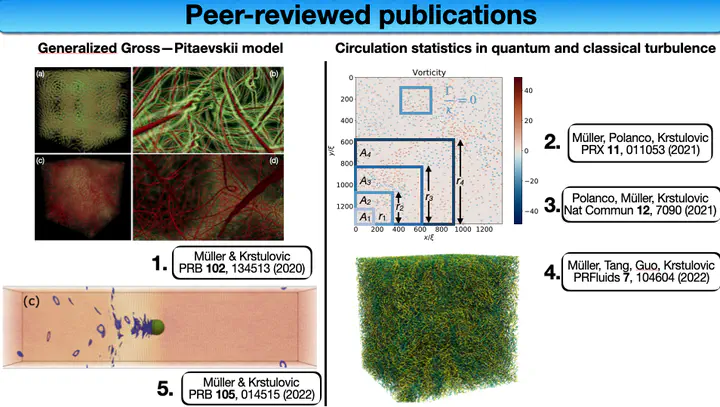# Nicolás P. Müller PhD Thesis## Quantum vortices, statistics of velocity circulation and excitations in superfluid turbulence

##### Université Côte d’Azur. December 14th 2022.

Superfluidity is a fascinating state of matter characterized by the absence of viscosity and the presence of topological defects with a quantized velocity circulation. These fundamental structures of the flow, also known as quantum vortices, interact and reconnect following very complex dynamics. The chaotic and disordered motion of these structures is known as quantum turbulence. They display rich multi-scale physics and some similarities with classical turbulence, such as the presence of a Kolmogorov-like energy cascade at large scales. Some typical examples of superfluids are Bose- Einstein condensates and superfluid helium at very low temperatures.

In this Thesis, we study numerically and analytically some statistical properties of quantum turbulence. We present a generalized Gross–Pitaevskii equation that takes into account strong and non-local interactions between bosons. In particular, this model allows us to consider the roton minimum in the excitation spectrum observed in superfluid helium. Performing extensive direct numerical simulations of this model, we address two specific problems: the study of quantum turbulence properties of the flow and the process of vortex nucleation and roton emission in the wake of an obstacle moving at different velocities.

The main scope of this Thesis is the study of velocity circulation statistics. This quantity, defined as the line integral of the velocity field around a closed loop, is a measure of the local rotation of the flow at a given scale. The goal is to characterize the intermittent nature of circulation statistics in quantum turbulence, provide a comparison with classical turbulence, and develop some analytical models to describe both systems. To achieve this, we analyze data from high-resolution direct numerical simulations of three models: the Gross–Pitaevskii equation for low-temperature quantum fluids, the Navier–Stokes equations for incompressible classical fluids, and a two-fluid model for finite-temperature superfluids. We also analyze data from superfluid helium experiments. Finally, we present some preliminary studies on velocity circulation in two-dimensional quantum turbulence.